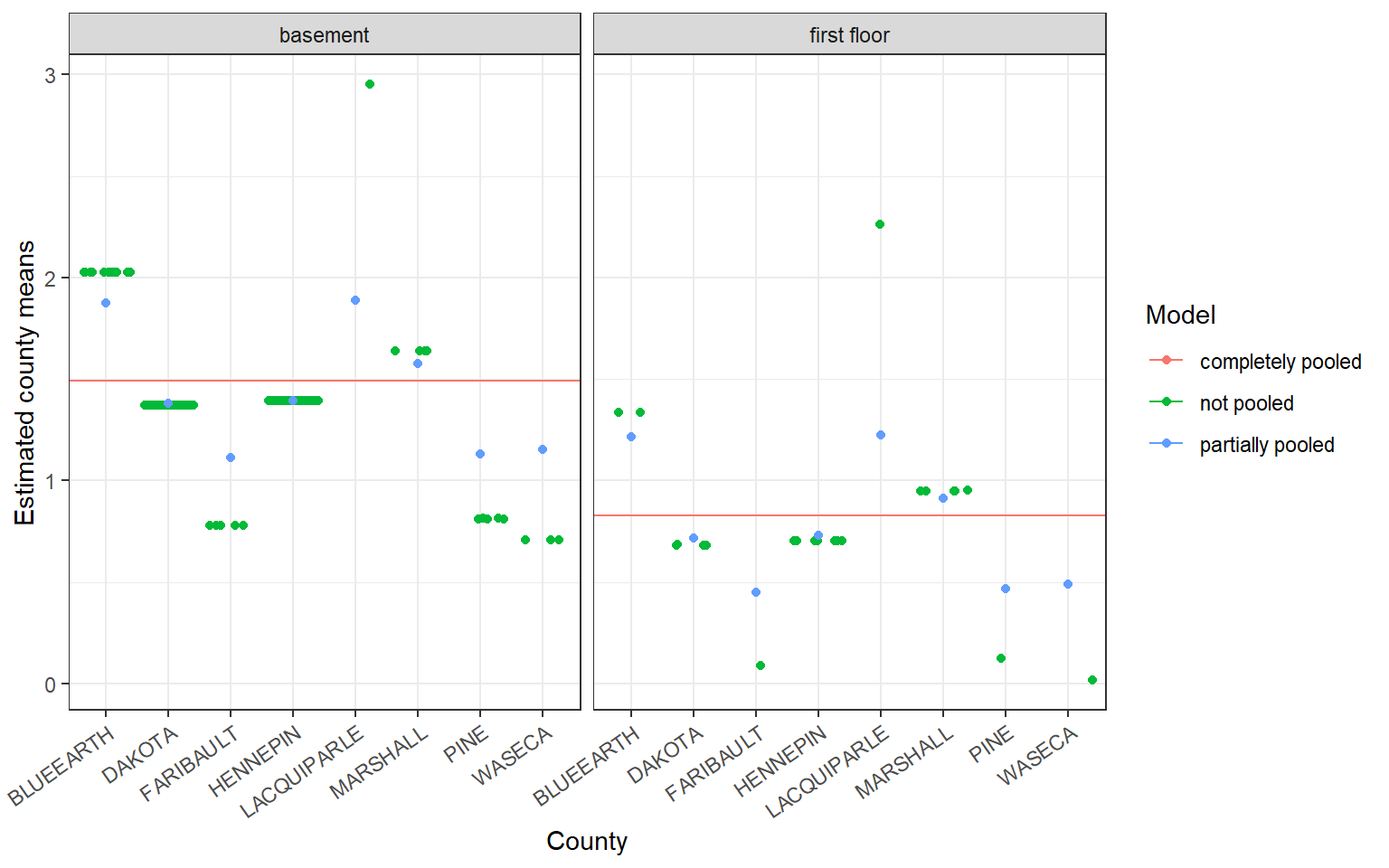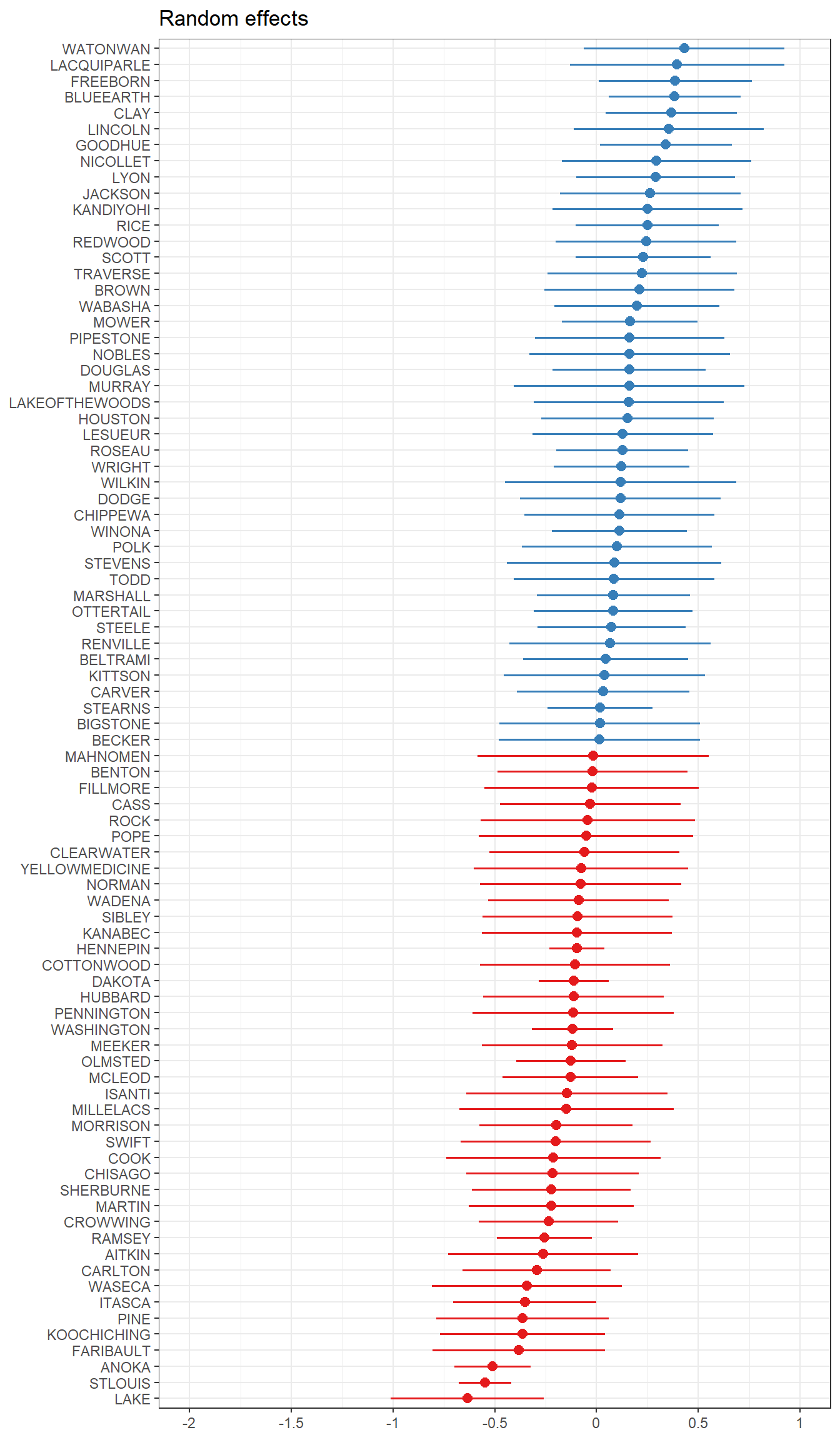## 17.6 Including Covariates

A similar sort of shrinkage effect is seen with covariates included in the model.

Consider the covariate $$floor$$, which takes on the value $$1$$ when the radon measurement was read within the first floor of the house and $$0$$ when the measurement was taken in the basement. In this case, county means are shrunk towards the mean of the response, $$log(radon)$$, within each level of the covariate.Covariates are fit using standard + notation outside the random effects specification, i.e. (1|county).

ri.with.x <- lmer(log_radon ~ floor + (1 |county), data=radon)
tab_model(ri.with.x, show.r2=FALSE)
Predictors Estimates CI p
(Intercept) 1.49 1.40 – 1.59 <0.001
floor [first floor] -0.66 -0.80 – -0.53 <0.001
Random Effects
σ2 0.53
τ00 county 0.10
ICC 0.16
N county 85
Observations 919

Note that in this table format, $$\tau_{00} = \sigma^{2}_{\alpha}$$ and $$\sigma^{2} = \sigma^{2}_{\epsilon}$$. The estimated random effects can also be easily visualized using functions from the sjPlot package.

plot_model(ri.with.x, type="re", sort.est = "(Intercept)", y.offset = .4)Function enhancements –

• Display the fixed effects by changing type="est".
• Plot the slope of the fixed effect for each level of the random effect sjp.lmer(ri.with.x, type="ri.slope") – this is being depreciated in the future but works for now. Eventually I’ll figure out how to get this plot out of plot_model().